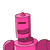# of a box of sweets is divided among 24 children, they will get 5 sweets each . How many would each get , if the number of children

of a box of sweets is divided among 24 children, they will get 5 sweets each . How many would each get , if the number of children is reduced by 4​

### 1 thought on “of a box of sweets is divided among 24 children, they will get 5 sweets each . How many would each get , if the number of children”

1.Each child gets = 5 sweets

24 children will get 24\times5=12024×5=120 sweets.

Total number of sweets = 120

If number of children is reduced by 4, then children left = 24 – 4 = 20

Now each child will get sweets = \frac{120}{20}=6

20

120

=6 sweets.

Hence, each sent will get 6 sweets.